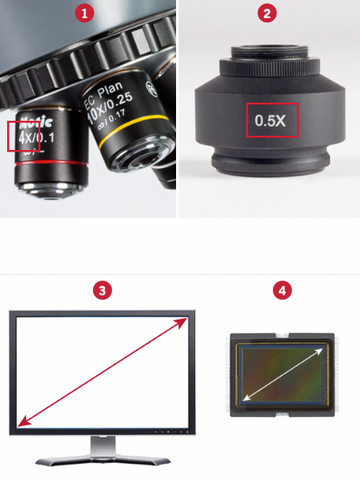# Magnification on Screen

### MAGNIFICATION ON SCREEN

One of the most frequently asked questions about digital imaging is: What is the total magnification showed on the screen?

To answer this question, we need to know four things:

(1) The objective’s magnification.
(3) The diagonal measurement of the screen
(in millimetres).
(4) The size of the camera sensor (in millimetres).The data for points (1) and (2), is easy to get, since it is usually engraved on each of these items.

The data for point (3), the diagonal measurement of the screen, is also easy to find out. Generally, manufacturers indicate the size of the screen in inches. We have to convert this measurement in millimetres. To do this, we have to multiply it by 25.4 and we will obtain our result. In case we do not know the size of the screen, we will need to measure it with a ruler or with measuring tape.

To obtain the data for point (4), we have to refer to the following table (5), because the real size of the sensor that is used for capturing the image differs a little bit from the size of the chip itself.Now that we have all the information we need, we will be able to find out the total magnification showed on the screen.

The formula is:

Total magnification =
Optical magnification x Digital magnification

The Optical magnification is:

The Digital magnification is:

(Screen size) / (Sensor size)

For example, if we are using a 40X objective with a 0.5X adapter, the camera sensor is 1/2” and the screen size is 14”, our calculations would be:

Optical magnification:

40 x 0,5 = 20

Digital magnification:

(14” x 25,4) 355,6 / 8 (as per table) = 44,45

TOTAL MAGNIFICATION:

20 x 44,45 = 889X

By The Motic Team | Motic Europe

Leave a note for our applications team if you are interested in learning more - either through a demo, a pricing quote or just additional brochures.